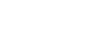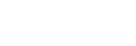Q1:Should we optimize the source of the QuEST or two contest cases random.c and GHZ_QFT.c?
Answer:The allowed modification to the source code excludes the provided circuit code. Because the provided circuit just like the calculating models, you can’t change it. Please do some optimizations related to the algorithm or others. But don’t change the provided circuit code, or the zero score will be got!

Q2:For the QuEST problem, we have known that some optimization techniques would cause error(for example, using GPU). May I ask for the final result, whether small error under a certain limit could tolerated or we need to keep the result precise?
Answer: The final results must be the exactly same as the referenced results. You should keep the answer precise. In this case, the GPU acceleration may cause some errors has no relationship to optimization.

Q3:We found there are various versions of QuEST. At present, we are using QuEST 3.0. Will it affect the verification of the output result?
Answer: “We strongly recommend using the stable version of QuEST_2.1.0 and the corresponding source code is available in Reference【2】”.You can see the last part of Application Background of the QuEST challenge in . Please read it carefully.

Q4:The QuEST has a latest version v3.0.1. We wonder whether we could use the latest version instead of v2.1.0, and if not could you tell us what's wrong in the program?
Answer:We still recommend the version 2.1.0 for this competition.The competition just focus on the optimization of algorithm for improving the performance of application. It doesn’t matter with version of application. We have done many tests using the version 2.1.0. It is fair to every participant using the same version of application for competition.

Q5:Can random and GHZ quantum circuits be simplified, decomposed, and then calculated?It's like simplifying the math and then calculating it.
Answer:The provided circuit (GHZ_QFT.cor random.c) can’t be modified. Any changes to the provided circuit is not allowed. Or you will get zero score.

Q6:Whether DeepSpeed can be used in the third question of ASC?
Answer:The DeepSpeed API is a lightweight wrapper on PyTorch. It will be allowed to be used.

Q7:In the task description you already mention various transformer networks. Are there any restrictions to this, i.e. are there any restrictions on the data we may use?
Answer:It is allowed to use pre-trained models such as BERT, ROBERTA.It is not forbidden to use additional datasets to train your model.

Q8:The test set does not give the correct answer. We can predict the answer, but how to calculate the score of Stest. We can calculate the model accuracy by the answer of dev set.
Answer:The score of Stest is the accuracy of test dataset

PartnersFollow us